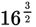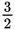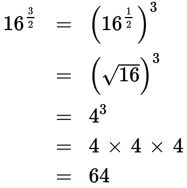# SAT Math Multiple Choice Question 285: Answer and Explanation

### Test Information

Question: 285

15. Which of the following representsas an integer?

• A. 4
• B. 12
• C. 48
• D. 64

Explanation:

D

Difficulty: Hard

Category: Passport to Advanced Math / Exponents

Strategic Advice: Because this is a non-calculator question, you need to rewrite the exponent in a way that makes it easier to evaluate. Unit fractions, as exponents, are easy to evaluate because they can be rewritten as radicals.

Getting to the Answer: Use the rules of exponents to rewriteas a unit fraction raised to a power. Then write the expression in radical form and simplify.